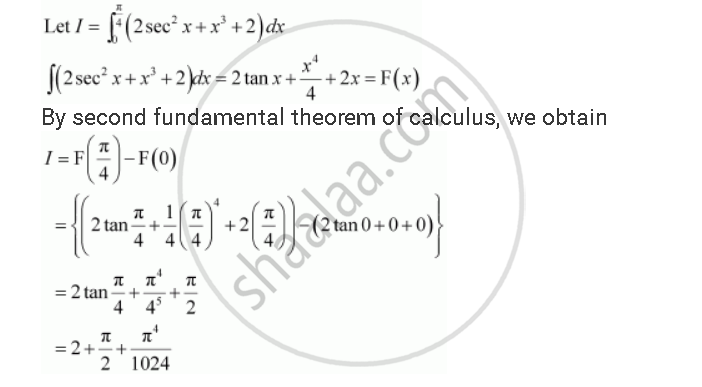Share

# Evaluate the Definite Integrals Int_0^(Pi/4) (2 Sec^2 X + X^3 + 2) Dx - CBSE (Commerce) Class 12 - Mathematics

#### Question

Evaluate the definite integrals int_0^(pi/4) (2 sec^2 x + x^3  + 2) dx

#### SolutionIs there an error in this question or solution?

#### Video TutorialsVIEW ALL 

Solution Evaluate the Definite Integrals Int_0^(Pi/4) (2 Sec^2 X + X^3 + 2) Dx Concept: Fundamental Theorem of Calculus.
S